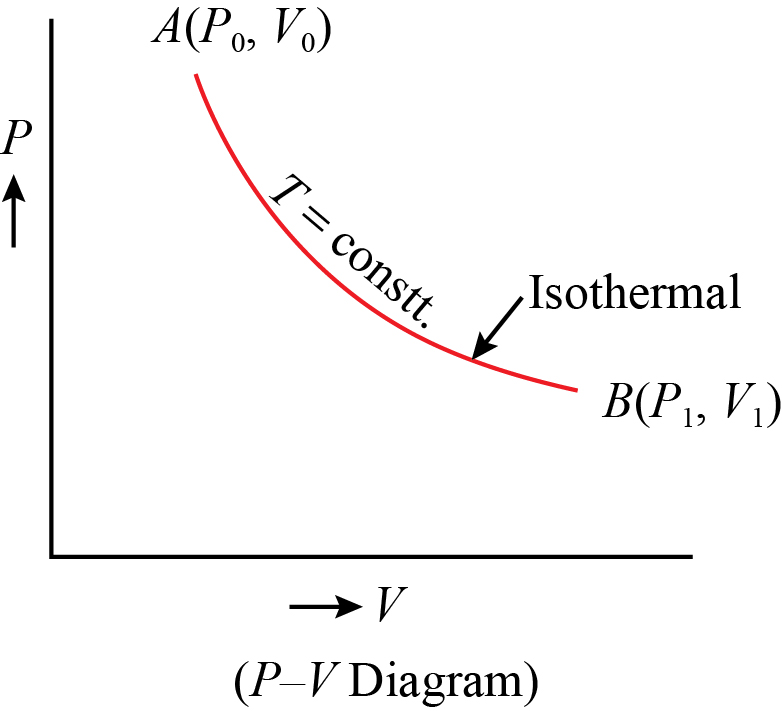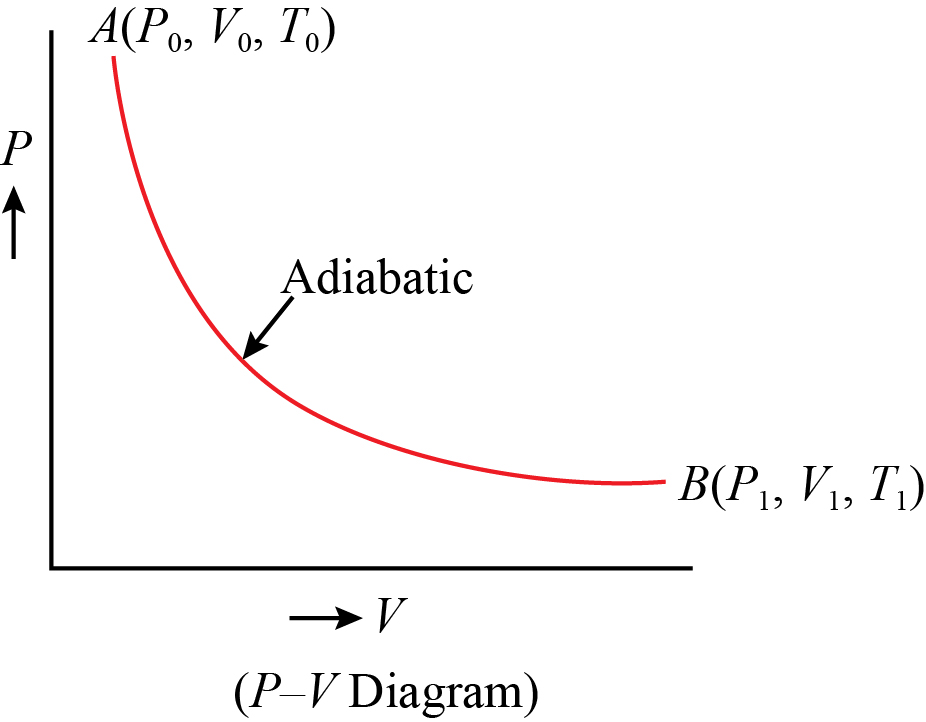Click to Chat

1800-1023-196

+91-120-4616500

CART 0

• 0

MY CART (5)

Use Coupon: CART20 and get 20% off on all online Study Material

ITEM
DETAILS
MRP
DISCOUNT
FINAL PRICE
Total Price: Rs.

There are no items in this cart.
Continue Shopping• Complete Physics Course - Class 11
• OFFERED PRICE: Rs. 2,968
• View Details

Work Done During Isothermal Expansion

Table of Content

Graphical Method

Analytical Method

Related Resources

Consider that one gram molecule of a perfect gas is taken in a cylinder having perfectly conducting walls and bottom, provided with a piston. Let the cylinder be placed on a source of heat at temperature T°A. If the piston is now moved slowly outwards, the gas expands, does some work and tends to cool but it absorbs required amount of heat from the source to keep it at the same temperature. The expansion is thus isothermal.

Let the piston of area of cross-section A move through a small distance dx so that the gas expands by a small amount dV. As the expansion is small, the pressure of the gas remains , practically, constant, say P.

Then the force acting on the piston, F = P×A

and small work done by the gas,

dW = F dx

= PA dx

dW = P dV        [Since, A dx = dV](a) Graphical Method

The isotherm PQ for the process is shown in below figure.

The net work done to expand the gas from a volume V1 to volume V2 will be,

W = Area ABDC

Thus, area occupied below the curve in between AB and CD gives the required value of work done.

(b) Analytical Method

Analytically, we can be calculated by integrating dW between the limits V1 to V2.

For a perfect gas, PV = RT or P = RT/V

Substituting P = RT/V in the above equation, we get,

As temperature is constant during isothermal expansion and R is a gas constant,

= RT (logeV2 -logeV1)

= RT loge (V2/V1)

W = 2.3026 RT log10 (V2/V1)

According to first law of thermodynamics, heat used up for the expansion of gas = W/J

So, H = 2.3026 (RT/J) log10 (V2/V1)

Since P1V1 = P2V2, thus, V2/V1= P1/P2

Substituting forV2/V1, H = 2.3026 (RT/J) log10 (P1/P2)

On the other hand, if the gas is compressed, the work is done on the gas and is therefore negative. The work done for isothermal compression is given by,

W = -2.3026 RT log10 (V2/V1)

So, W =2.3026 RT log10 (V1/V2)

Therefore, H = 2.3026 (RT/J) log10 (V1/V2)

and H = 2.3026 (RT/J) log10 (P2/P1)

Problem 1:-

Calculate the work done of n moles of a vander Waals gas in an isothermal expansion from Vi to Vf.

Concept:-

The work done W of gas in an isothermal expansion from volume Vi to Vf is defined as,

W = -∫ViVf p dV

The gas equation for van der Wall gas is,

(p+n2a/V2) (V-nb) = nRT

Here p is the pressure, V is the volume, R is the gas constant, T is the temperature, n is the number of moles, a and b are the van der Walls gas constant.

Solution:-

First we have to find out the pressure p of the gas.

From van der Walls gas equation (p+n2a/V2) (V-nb) = nRT, the pressure p will be,

p = (nRT/(V-nb)) – (n2a/V2)

To obtain the work done of n moles of a van der Walls gas in an isothermal expansion from volume Vi to Vf will be,

W = -∫ViVf p dV

= -∫ViVf [((nRT/(V-nb)) – (n2a/V2)] dV

= [- nRT ln(V-nb) – an2/V] ViVf               (Since, ∫ (1/ V-nb) dV = ln(V-nb) and ∫ 1/V2 dV = - 1/V)

= (- nRT ln Vf –nb/ Vi –nb) –an2(1/Vf- 1/Vi)

From the above observation we conclude that, the work done of n moles of a van der Walls gas in an isothermal expansion from volume Vi to Vf would be (- nRT ln Vf –nb/ Vi –nb) –an2(1/Vf- 1/Vi).

Problem 2:-

A quantity of ideal gas occupies an initial volume V0at a pressure p0 and a temperature T0. It expands to volume V1 (a) at constant pressure, (b) at constant temperature, and (c) adiabatically. Graph each case on a pV diagram. In which case is Q greatest? In which case is W greatest? least? In which case is ?Eint greatest? Least?

Concept:-

Isobaric Process:-

The process in which, the change in volume and temperature of a gas takes place at a constant pressure is called an isobaric process.

Isothermal Process:-

The process in which, the change in pressure and volume takes place at constant temperature is called an isothermal change.

The process in which, the change in pressure and volume and temperature takes place without any heat entering or leaving the system is called adiabatic change.

Solution:-

(a) Isobaric Process:-

The process in which, the change in volume and temperature of a gas takes place at a constant pressure is called an isobaric process.

A quantity of ideal gas occupies an initial volume V0 at a pressure p0 and a temperature T0. It expands to volume V1 at constant pressure. The pV diagram for this process as shown in below figure1.

Figure 1:(b) Isothermal Process:-

The process in which, the change in pressure and volume takes place at constant temperature is called an isothermal change.

A quantity of ideal gas occupies an initial volume V0 at a pressure p0 and a temperature T0. It expands to volume V1 at constant temperature. The pV diagram for this process as shown in below figure 2.

Figure 2:The process in which, the change in pressure and volume and temperature takes place without any heat entering or leaving the system is called adiabatic change.

A quantity of ideal gas occupies an initial volume V0 at a pressure p0 and a temperature T0. It expands to volume V1 adiabatically. The pV diagram for this process as shown in below figure 3.

Figure 3:Q is greatest for constant pressure process and least for adiabatic. W is greatest (in magnitude, it is negative for increasing volume process) for constant pressure process and least for adiabatic. ΔEint is greatest for constant pressure (for which it is positive), and least for adiabatic (for which is negative).

Problem 3:-

A sample of gas expands from 1.0 to 5.0 m3 while its pressure decreases from 15 to 5.0 Pa. How much work is done on the gas if its pressure changes with volume according to each of the three processes shown in the pV diagram in below figure.Concept:-

Work is a path function. So work done on the gas depends upon the path. The area under pv diagram gives the work done on the gas between initial state i and final state f.

Work done W is defined as,

W = -pdV

Here p is the pressure and dV is the change in volume.

Solution:-

(1) In the process 1, the work done W1 will be zero for vertical path.

So W1 = 0

The work done is present only in horizontal path.

Thus the work done W2 for horizontal path will be,

W2= -pdV     (negative sign is due to work is done on the gas

= - (15 Pa) (4m3)

= -60 Pa.m3

= (-60 Pa.m3) (1 J/1 Pa.m3)

= -60 J

Therefore the net work done W on the gas will be,

W = W1 + W2

= 0 J+(-60 J)

= -60 J

From the above observation we conclude that, the net work done on the gas would be -60 J.

(2) For the process 2, work done is negative of the area under the curve.

So the area A under the curve for process 2 will be,

A = ½ (15 Pa+5 Pa) (4m3)

= 40 Pa.m3

= (40 Pa.m3) (1 J/1 Pa.m3)

= 40 J

As for the process 2, work done is negative of the area under the curve; therefore the net work done on the gas will be -40 J.

(3) In the process 3, the work done W3 will be zero for vertical path.

So W3 = 0 J

The work done is present only in horizontal path.

Thus the work done W4 for horizontal path will be,

W4= -pdV     (negative sign is due to work is done on the gas)

= - (5 Pa) (4m3)

= -20 Pa.m3

= (-20 Pa.m3) (1 J/1 Pa.m3)

= -20 J

Therefore the net work done W on the gas will be,

W = W3 + W4

= 0 J+(-20 J)

= -20 J

From the above observation we conclude that, the net work done on the gas would be -20 J.

Conceptual Question

Real gases always cool when making a free expansion, whereas an ideal gas does not. Explain.

Solution:-

In a free expansion of an ideal gas, the gas is initially in one side of the container, and when the stopcock is opened, the gas expands into the previously evacuated half. No weights can be raised in this process results no work is done. The process is adiabatic since the container is insulated. Thus W = 0 and Q = 0. So, according to first law of thermodynamics the internal energy will be zero.

The internal energy of an ideal gas undergoing a free expansion remains constant. This signifies that the temperature of the ideal gas does not change in a free expansion. But the internal energy of a real gas undergoing a free expansion does not remain constant results a change in temperature will occur in the expansion of real gas. That is why real gases always cool when making a free expansion, whereas an ideal gas does not.

Related Resources

Look into the Sample Papers of Previous Years to get a hint of the kinds of questions asked in the exam.

To read more, Buy study materials of Thermodynamics comprising study notes, revision notes, video lectures, previous year solved questions etc. Also browse for more study materials on Chemistry here.### Course Features

• 101 Video Lectures
• Revision Notes
• Previous Year Papers
• Mind Map
• Study Planner
• NCERT Solutions
• Discussion Forum
• Test paper with Video Solution